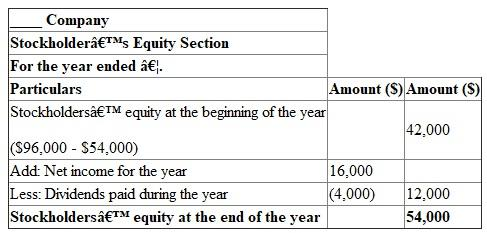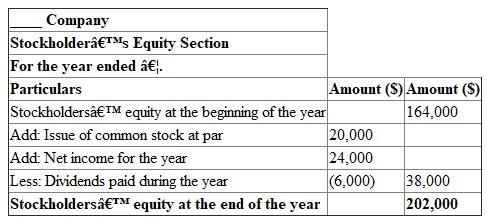# Quiz 2: Financial Statements and Accounting Conceptsprinciples

Business

Stockholder's equity at the end of the year: The stockholder's equity at the end of the year is the balance of beginning equity and the retained earnings payable to the stockholders. The stockholders equity at the end of the year is calculated as the sum of beginning stockholder's equity and the net income, as reduced by the dividends paid for the year. Use the following table to calculate the stockholder's equity at the end of the year:Hence, the stockholder's equity at the end of the year is \$54,000.

Stockholder's equity at the end of the year: The stockholder's equity at the end of the year is the balance of beginning equity and the retained earnings payable to the stockholders. The stockholders equity at the end of the year is calculated as the sum of beginning stockholder's equity and the net income, as reduced by the dividends paid for the year. Use the following table to calculate the stockholder's equity at the end of the year:Hence, the stockholder's equity at the end of the year is \$202 ,000.

Calculate the cost of goods sold: The cost of goods sold is the difference between the net sales and the gross profit. It is given that the net sales are \$250,000 and the gross profit is \$100,000. Use the following formula to calculate the cost of goods sold:Hence, the cost of goods sold is \$150,000. Calculate the income from operations: Income from operations is the difference between gross profit and operating expenses. Operating expenses include selling, general and administrative expenses. It is given that the gross profit is \$100,000 and the selling, general and administrative expenses are \$44,000. Use the following formula to calculate the income from operations:Hence, the income from operations is \$56,000. Calculate income before taxes: Income before taxes is the sum of net income and the income tax expense. It is given that the net income is \$40,000 and the income tax expense is \$10,000. Use the following formula to calculate the income before taxes:Hence, the income before taxes is \$50,000. Calculate the interest expense: Interest expense is the difference between the income before taxes and the income from operations. We calculated the income from operations is \$56,000 and the income before taxes is \$50,000. Use the following formula to calculate the interest expense:Hence, the interest expense is \$6,000.